Improving economics teaching and learning for over 20 years

# Interactive Graphs for Statistics for Social Science

### Martin Poulter, DeSTRESS Project

These graphs redraw themselves dynamically in response to user clicks (or touches, if you're using a tablet device). They are web pages with embedded programs, requiring no plug-ins or special software. They may be quite slow in some versions of Internet Explorer.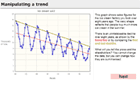1. Effect on trendlines of censoring data
regression line; effect of choosing starting point2. Evaluating the Rule of 70
exponential growth; percentage error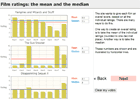3. Mean versus median: film ratings
robustness of median relative to the mean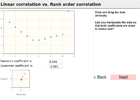4. Pearson versus Spearman correlations
types of correlation; similarities and differences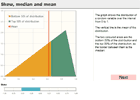5. Skew, mean and median
mode, mean, median of continuous (triangular) distribution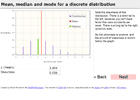6. Skew in a discrete distribution (counterpoint to above)
mode, mean, median of discrete (Poisson) distribution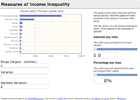7. Measures of income spread
absolute and percentage change; range, s.d. and variance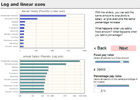9. Logarithmic axes and income
measures of spread; absolute change versus percentage change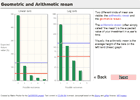10. Arithmetic versus geometric mean
probability; expected return; geometric mean as long-term expected return11. The shape of the sampling distribution
sampling from a skewed distribution; distinguishing the population, sample and sampling distribution

12. Distribution of sample standard deviations and why we use n-1 to calculate sample sd (incomplete)
sampling; Bessel's correction

13. Correlation of a sample (incomplete)

Licences: Creative Commons Attribution-ShareAlike (text content); MIT licence (Javascript code).

### University of Sussex